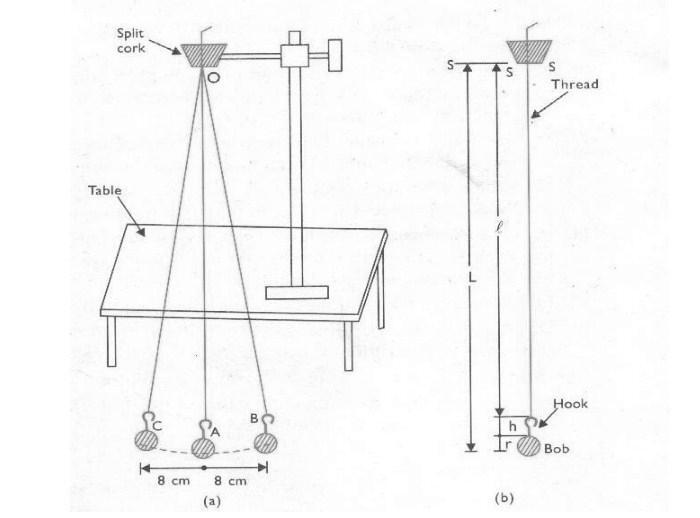# To Determine the Value of g using Simple Pendulum

## Objective / Aim of the Experiment

To determine the value of ?g? using simple pendulum.

### Apparatus / Equipment Required

A metallic spherical bob with a hook
Clamps stand
Split cork
Stop clock / watch
Verneir Calipers
Meter scale
Chalk piece

### Formula used

(i) T = 2 (l / g)
where, T = time period of oscillations
l = effective length of pendulum
g = acceleration due to gravity

(ii) A second a pendulum is the pendulum whose oscillation period is 2s

### Procedure

1. Take a vernier calipers and find its vernier constant. Also find its zero error, if any, with its proper sign.

2. Measure the diameter of given metallic bob along different axes and find the mean diameter of bob. Apply appropriate zero correction and determine the corrected diameter of the bob. Let it be d. Then find radius of bob r = D/2

3. Either using vernier calipers or a meter scale note the length of the hook attached to the pendulum bob let it be h.

4. Take a then inextensible but strong cotton thread of length about 2m. Tie one end of the thread tightly to the hook of the bob. Pass other end of the thread through the two split half pieces of cork. Hold the cork firmly in the clamp stand and ensure that the cut of cork piece is at right angle to the edge of the table.

5. Place the stand on the table/stool, in such a way that the pendulum bob is just 2-3cm above the floor of laboratory.

6. Mark a line, with a piece of chalk, just below the pendulum bob and parallel to the edge of the table. Mark a point A just below the bob in its equilibrium poison and two other points B and C on mutually opposite sides of point A on the line at a distance of about 8-10 cm.

7. Adjust the length of thread below the cork piece so that sum of the length of thread + length of hook + radius of pendulum bobs.

8. Displace the pendulum bob by hand from its mean poison. A to the marked point B on the line parallel to the edge of the table. Gently release it so that the bob starts vibrating to and fro about its mean position. A along the starlight line ABC and there is no spinning motion of the pendulum bob, If it is so then it is alright.

9. Note the least count of your stop clock/watch. also note zero error, if any present in the stop clock/watch with its proper sign. Then find the value of zero correction. Zero correction is negative of the zero error.

10. Just like step 8 displace the bob to mark B on line marked and gently release it so that it starts vibration to and fro. Start the stop clock/watch.

11. Observe the motion of pendulum bob. After starting of stop clock, the bob goes to point B, returns to its mean position A, then goes to left extreme position C and finally returns back to mean position A and again start moving start moving towards right, count 1.

12. In this way let the pendulum completes 19 vibrations. Now be alert. as soon as the pendulum complete and find the corrected time taken by the pendulum to complete 20 vibrations.

13. Increase the effective length of pendulum in steps o f 10 cm each to 90 cm, 100 cm.............. and repeat th steps 10-13 every time so as to measure the corresponding values of time token by the pendulum for 20 vibrations for each length.

14. In the manner take readings for pendulum to complete 20 vibrations.

15. Dividing the values of times for 20 vibrations by 20 determine the value of time period T of pendulum corresponding to different lengths. Also find the values of T square.### Observation

Vernier constant of vernier calipers (V.C.) = ................... cm.

Zero error of vernier calipers (with sign) = ................... cm.

Zero error correction of vernier calipers (with sign) = ................... cm.

Observed diameter of the pendulum bob
(i) ................... cm
(ii) ................... cm
(iii) ................... cm.

Mean value of observed diameter of pendulum bob = ................... cm.

Corrected diameter of pendulum bob D = ................... cm.

Radius of pendulum bob r = ................... cm.

Length of the hook of pendulum bob h = ................... cm.

Least count of stop clock/watch= ................... s.

Zero error present in stop clock ................... s.

Zero correction in stop clock ................... s.

### Calculations

1. From the observed data plot a graph by taking length of pendulum L along X-axis and the time period of oscillation T along Y-axis. The graph obtained is is parabolic.

2. Plot a graph by taking length of pendulum L along X-axis and T squre along Y-axis. The graph obtained is shown; the graph is a straight line inclined to either axes. It shows that: T square propositional to the L

3. From L-T square graph determine the length of the pendulum corresponding to T square = 4 s square the time period of pendulum is T = 2. This length corresponds to the length of the seconds pendulum.

### Precaution

• The thread used should be weightless ,strong and inextensible.
• The point of suspension should be fixed. It is possible when the clamp stand is rigid.
• Lower faces of split cork should be in same level.
• Length of the pendulum should be counted from the point of suspension to the centre mass of the pendulum bob.
• The pendulum bob should vibrate should be small.

## Related Categories### Spreading Knowledge Across the World

USA - United States of America  Canada  United Kingdom  Australia  New Zealand  South America  Brazil  Portugal  Netherland  South Africa  Ethiopia  Zambia  Singapore  Malaysia  India  China  UAE - Saudi Arabia  Qatar  Oman  Kuwait  Bahrain  Dubai  Israil  England  Scotland  Norway  Ireland  Denmark  France  Spain  Poland  and  many more....